Using Decimals - Converting to mm, cm, km, kg, rupees

Chapter 8 Class 6 Decimals
Concept wise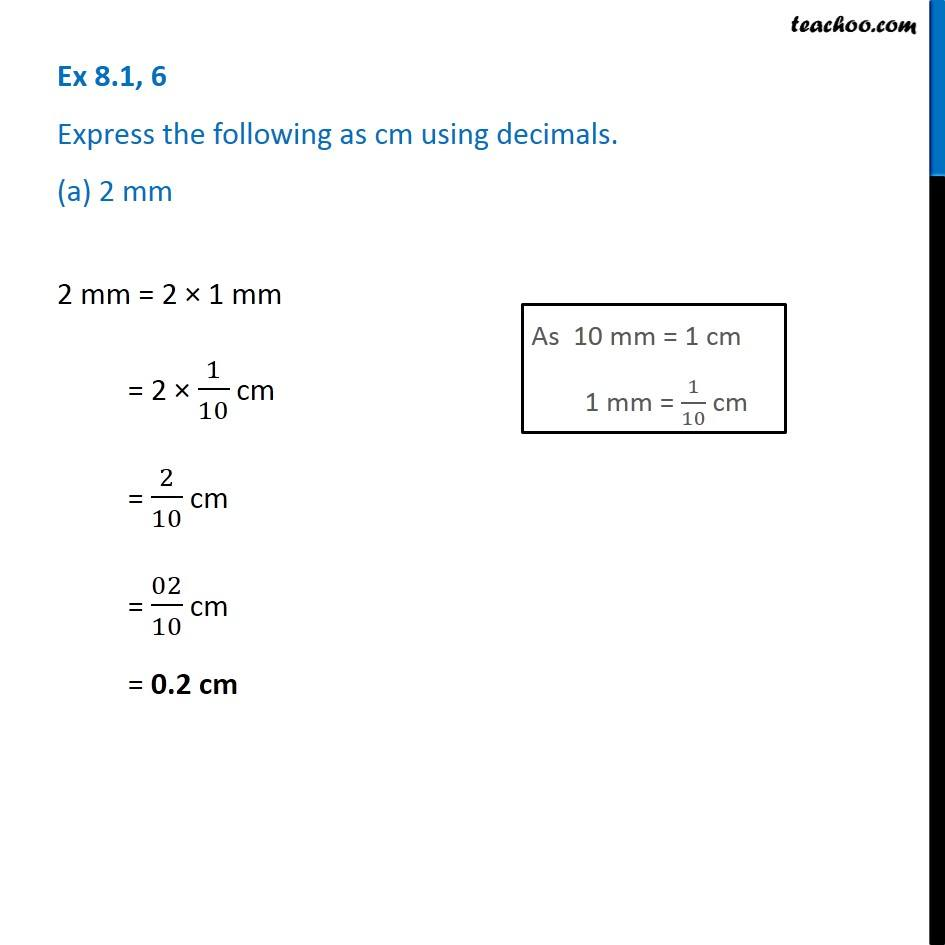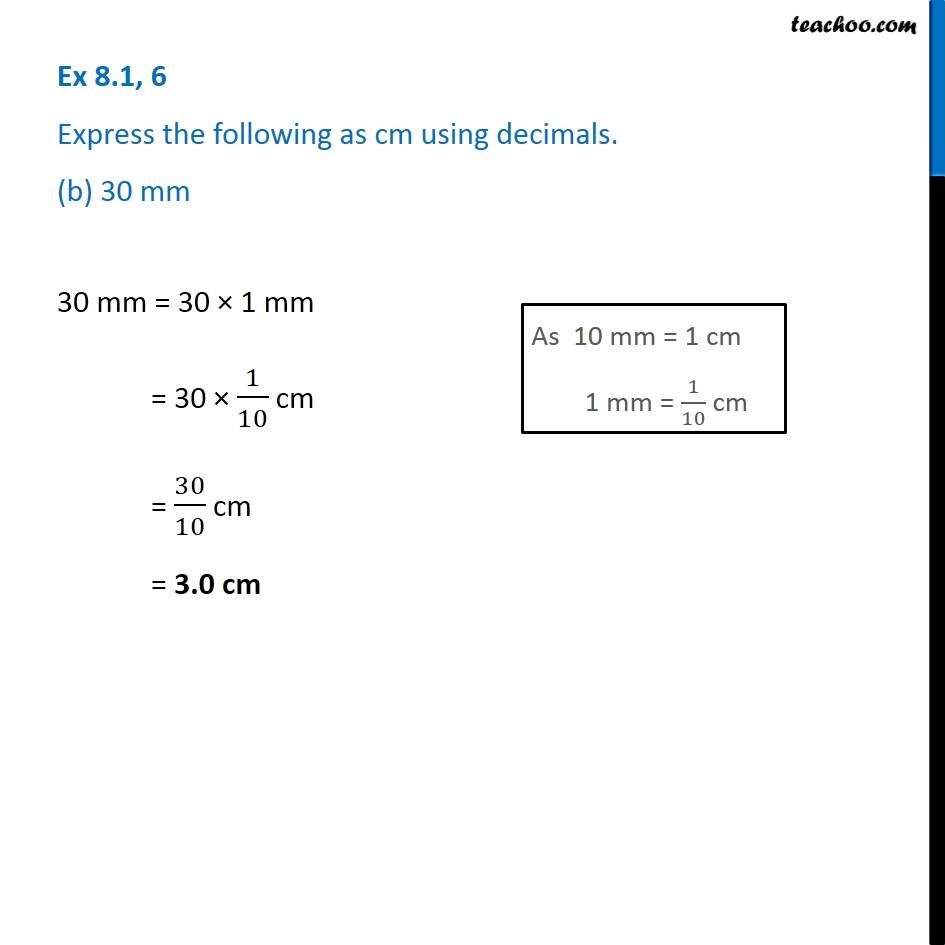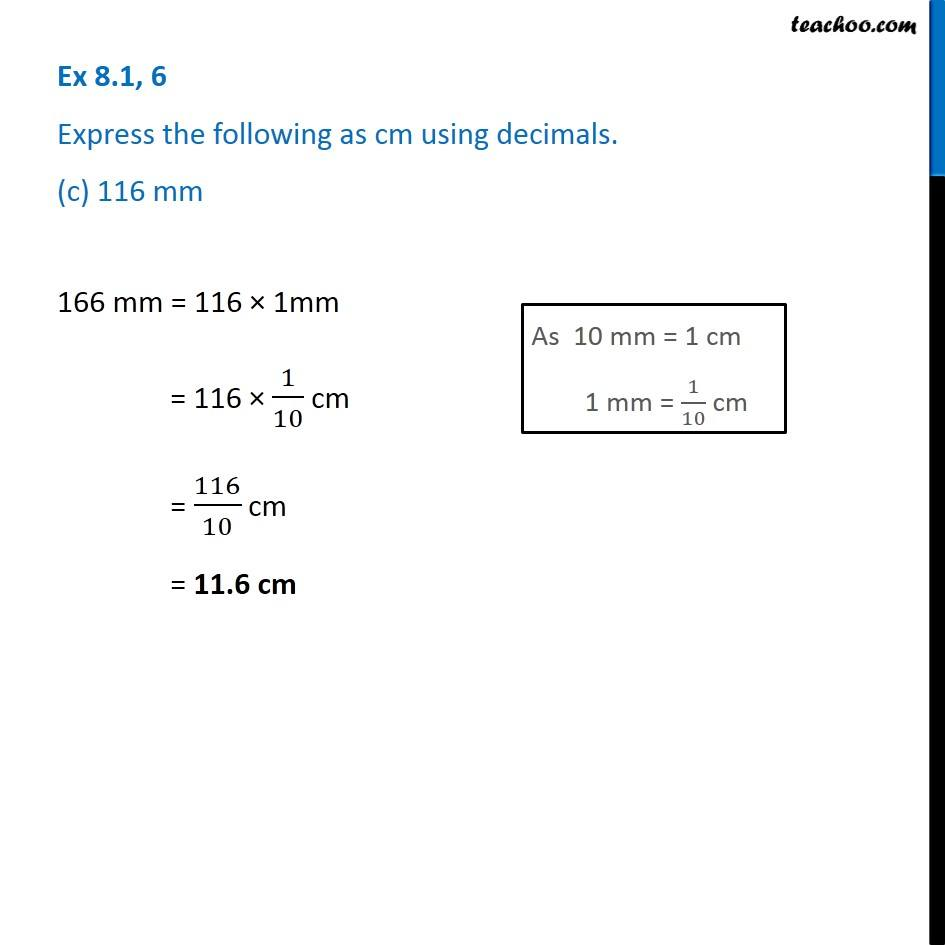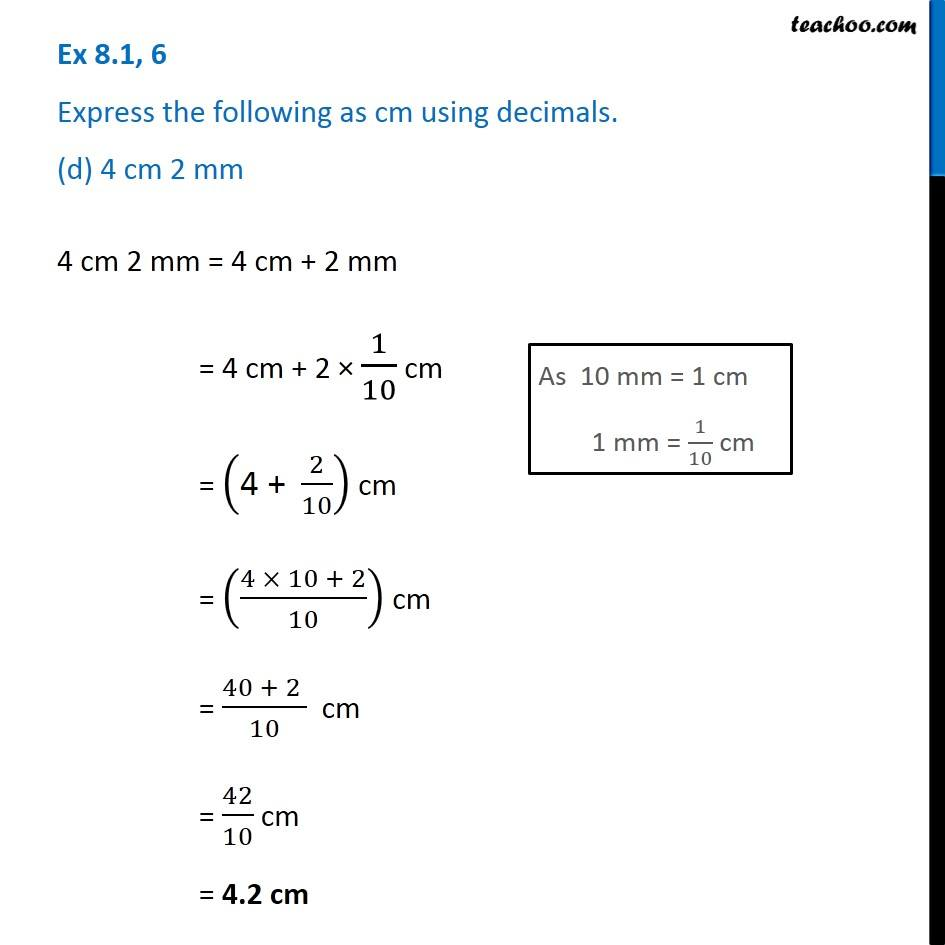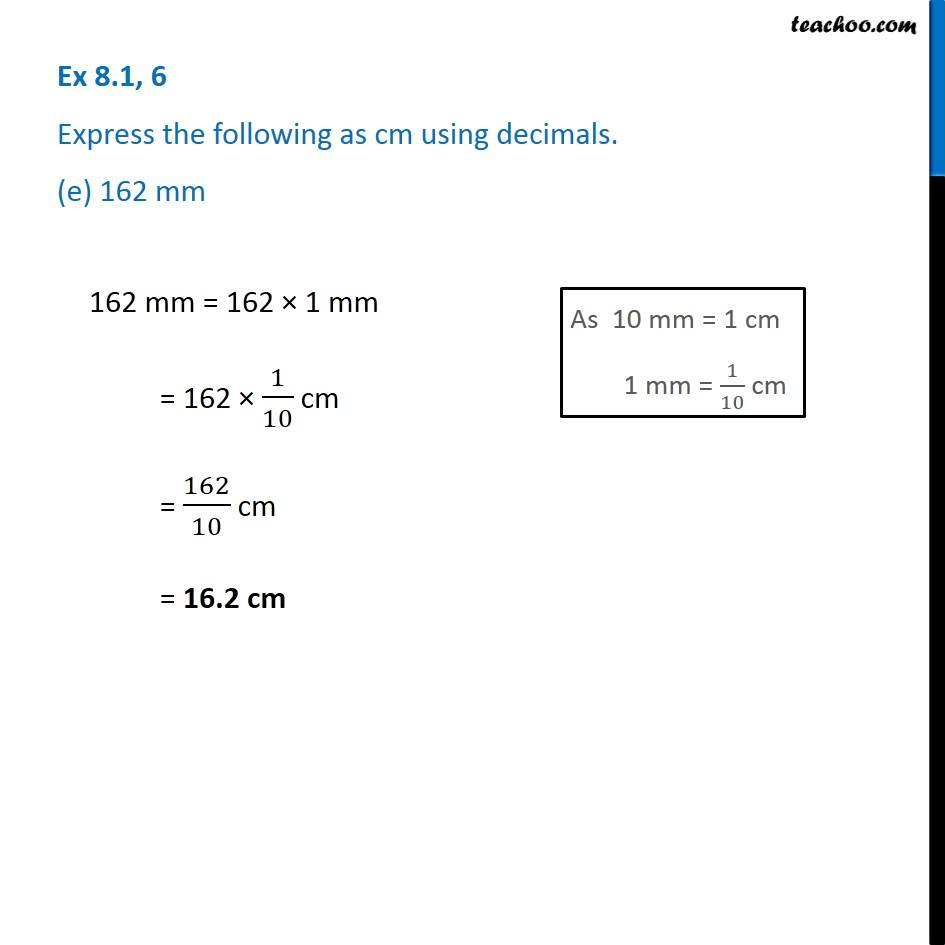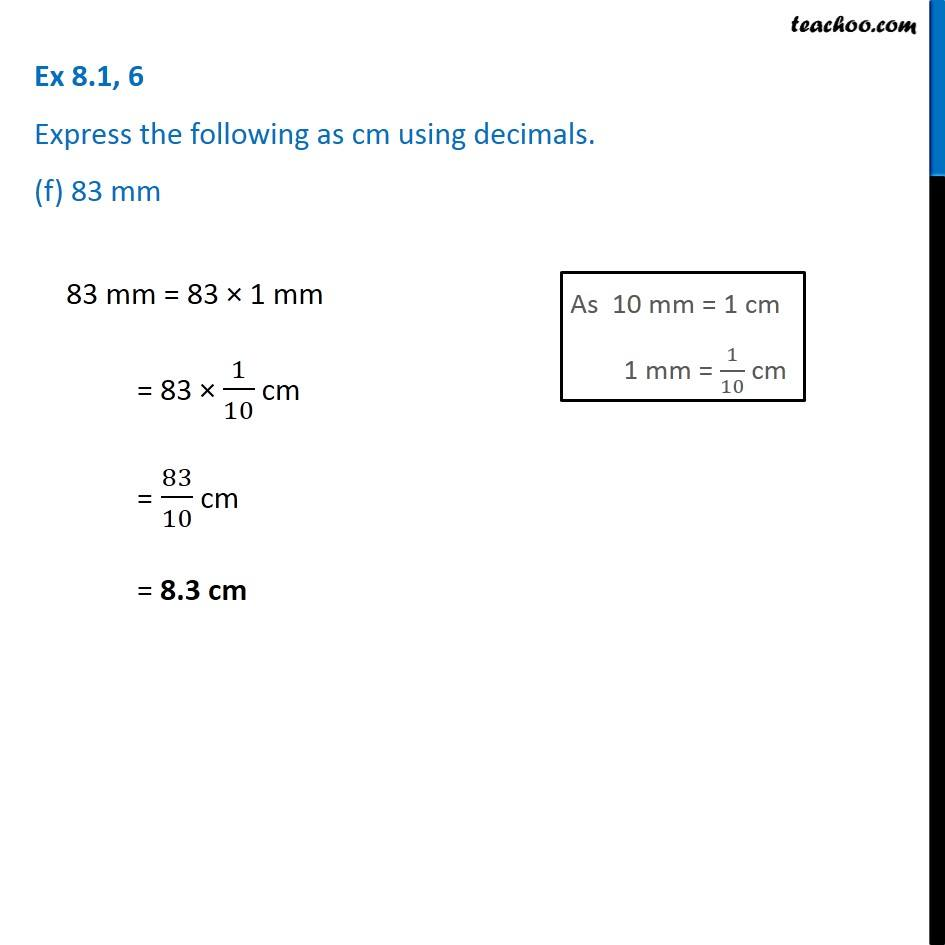### Transcript

Ex 8.1, 6 Express the following as cm using decimals. (a) 2 mm 2 mm = 2 1 mm = 2 1/10 cm = 2/10 cm = 02/10 cm = 0.2 cm Ex 8.1, 6 Express the following as cm using decimals. (b) 30 mm 30 mm = 30 1 mm = 30 1/10 cm = 30/10 cm = 3.0 cm Ex 8.1, 6 Express the following as cm using decimals. (c) 116 mm 166 mm = 116 1mm = 116 1/10 cm = 116/10 cm = 11.6 cm Ex 8.1, 6 Express the following as cm using decimals. (d) 4 cm 2 mm 4 cm 2 mm = 4 cm + 2 mm = 4 cm + 2 1/10 cm = ("4 + " 2/10) cm = ((4 10 + 2)/10) cm = (40 + 2 )/10 cm = 42/10 cm = 4.2 cm Ex 8.1, 6 Express the following as cm using decimals. (e) 162 mm 162 mm = 162 1 mm = 162 1/10 cm = 162/10 cm = 16.2 cm Ex 8.1, 6 Express the following as cm using decimals. (f) 83 mm 83 mm = 83 1 mm = 83 1/10 cm = 83/10 cm = 8.3 cm